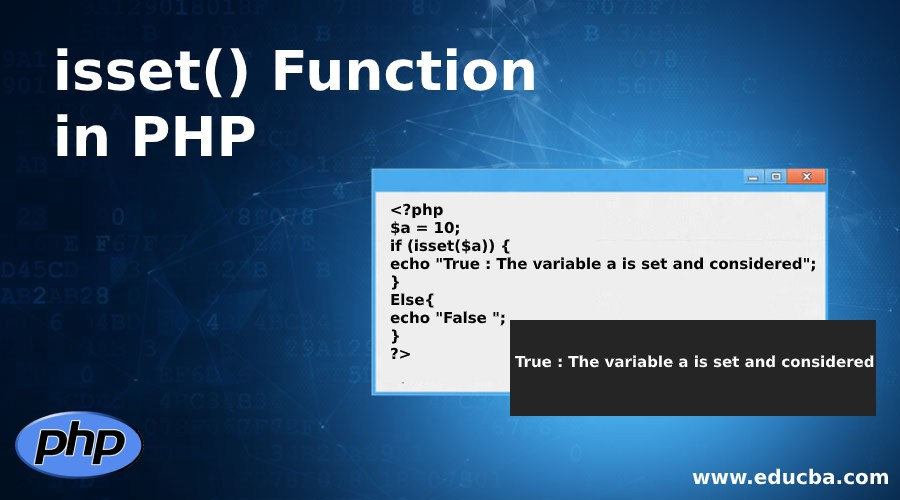## Introduction to isset() Function in PHP

The isset() function of PHP usually checks whether variable is set/declared and it is different/other than the NULL. If the variable is unset using the unset() function in PHP then it is no need to be considered at all to set. The isset() function will return the value TRUE only if the variable existed which is not NULL. Otherwise isset() function will return the value FALSE value when the function will check the variable which is assigned to the NULL term. NULL character “\0” is not at all equivalent to the PHP NULL constant term. If multiple terms passed to isset() then it will return TRUE value if all the parameters considered.

#### Syntax:

`Isset(\$variable, ……. );`

Explanation:

• \$variable: A variable is required which is specified to check
• ……. : It is an optional value/values or variable/variables to check

### How does isset() Function works in PHP

The isset() function works by returning the value TRUE if the \$variable exists in the isset() function (isset() programming code) which has the value which is other than NULL value. It is FALSE otherwise. The isset() function works from the PHP version 4.0. The return type of the isset() function of PHP programming language is Boolean. If the PHP isset() function are passed with the multiple variables then isset() will TRUE only if all the variables are set. The variable of the isset() function can be unset using the unset() function. Isset() function also works by accepting the multiple variables/many variables etc.. From the PHP 5.4.0 version, non-numeric offsets of strings will return FALSE value/values.

### Examples to implement isset() Function in PHP

Below are the examples:

#### Example #1

Code:

```<?php \$a = 10; if (isset(\$a)) { echo "True : The variable a is set and considered"; } Else{ echo "False "; } ?>```

Output: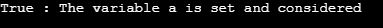Explanation: In the above isset() program, a variable ‘a” is declared and also defined with the value “10”. Then an isset() function inside the IF condition is made to return the value TRUE or FALSE but here the variable “a” is defined so the output is obviously “TRUE”. If the isset() function will return/print the value “FALSE” if the isset() returns FALSE value.

#### Example #2

Code:

```<?php \$a = 20; if (isset(\$a)) { echo "The Variable 'a' is now set.<br>"; } else { echo "The Variable 'a' is now unset.<br>"; } \$b = null; if (isset(\$b)) { echo "The Variable 'b' is now set.<br>"; } else { echo "The Variable 'b' is now unset.<br>"; } if (isset(\$c)) { echo "The Variable 'c' is now set.<br>"; } else { echo "The Variable 'c' is now unset.<br>"; } ?>```

Output: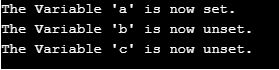Explanation: In the above example, a new variable “a” is created using with the value “10”. It means the value is set for the variable a. So the isset(\$a) will return TRUE value. If the IF condition of TRUE value then the statements inside the IF condition will be printed. It will print “The variable ‘a’ is now set”. If the IF condition returns FALSE value then the ELSE condition’s statements will be printed. Then variable “b” is created by assigning the NULL value. So the “isset(\$b)” will return the “FALSE” value. It meanse If(FALSE) will print the statements which are inside the ELSE condition which is “The Variable ‘b’ is now unset” because IF condition is FALSE and gone to the ELSE condition.

Now the isset(\$c) is placed inside the IF condition but the variable “\$c” is not assigned with any value so the value of the “\$c” is considered as NULL/FALSE value. So the IF condition’s value becomes FALSE and bypasses the IF condition and goes to the ELSE condition and prints the statements which are in the ELSE condition. It will print “The variable ‘c’ is now unset”.

#### Example #3

Code:

```<?php \$a1=51; \$b1=61; \$c1=NULL; if(isset(\$a1,\$b1,\$c1)){ echo "Here All variables are now set."; } else{ echo "Here All or Any variables are now Unset."; } ?>```

Output:Explanation: In the above example, variable’s “\$a1”, “\$b1”, “\$c1” variables are created with the values “51”, “61” and “NULL”. Here multiple variables are checked whether all the values to the variable’s are assigned or not. Here isset (\$a1,\$b1,\$c1) inside of the IF condition returns the FALSE value because the variable “\$c1” value is declared as the value “NULL” so the ELSE condition’s statements will be printed. It will print “Here All or Any variables are now Unset”. You can add as many variables as needed inside isset() function to check whether they are declared/set or undeclared/unset/NULL.

#### Example #4

Code:

```<?php \$var11 = 'test1'; \$var21 = 'another test2'; if (isset(\$var11) && isset( \$var21)) { echo "Now It is going to print because all variables are now set. </br>"; echo "==> 1. checking the var11 using isset():::</br>"; var_dump (isset(\$var11)); echo "</br></br>==> 2. checking the var21 using isset():::</br>"; var_dump (isset(\$var21)); } unset (\$var11); unset (\$var21); echo "</br> </br>The Variables which are after the unset:: </br>"; var_dump (isset(\$var11)); var_dump (isset(\$var21)); ?>```

Output: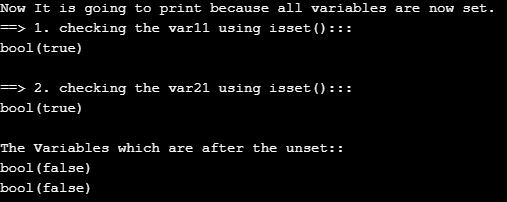Explanation: In the above example, isset() and unset() functions are used in the PHP programming language. The Variables “\$var11” and “var21” are created with the values “test1” and “another test2”. The values can either be a string value or integer value or any other etc. So the isset(\$var11) and isset(\$var21) will return the value TRUE. So the IF condition will return TRUE and prints the statements which are present inside the IF condition. Var_dump() is used to check whether the isset(\$var11) and isset(\$var21) is TRUE or not. Then again unset() function is used to unset the values of \$var11 and \$var21 variables. Now again checked the isset(\$var11) and isset(\$var21) values using the var_dump() function and it will return the value FALSE “bool(false)”. You can check the output of the above example to understand the unset() concept better using the image in the output section.

#### Example #5

Code:

```<?php \$user1 = 'pavankumarsake'; \$_SESSION['userid1'] = \$user1; if (isset(\$_SESSION['userid1'])) { echo " Here the Session is now available, Welcome to the \$_SESSION[userid1] "; } else { echo " Here No Session is available, so please Login "; } ?>```

Output: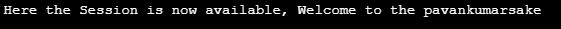Explanation: This is the example to check whether the session variable is available or not using the isset() function. Here “\$user1” is created and assigned a string value “pavankumarsake”. Then session id is created and assigned the \$user1 variable to it. So the isset(session variable) will return TRUE value and the IF condition becomes TRUE and print the statements which are inside the IF condition. If the IF condition returns False then the else statements will be printed. Else statements will be printed only if the \$user1 value is not defined/declared or declared with the NULL value.

### Conclusion

I hope you understand what is the definition of isset() function in PHP and it’s syntax, How isset() function works using PHP along with the various examples to understand the concept of isset() function.

### Recommended Articles

This is a guide to isset() Function in PHP. Here we discuss Syntax to isset() Function in PHP, how does it work,examples with codes and outputs. You can also go through our other related articles to learn more –

The post isset() Function in PHP appeared first on EDUCBA.